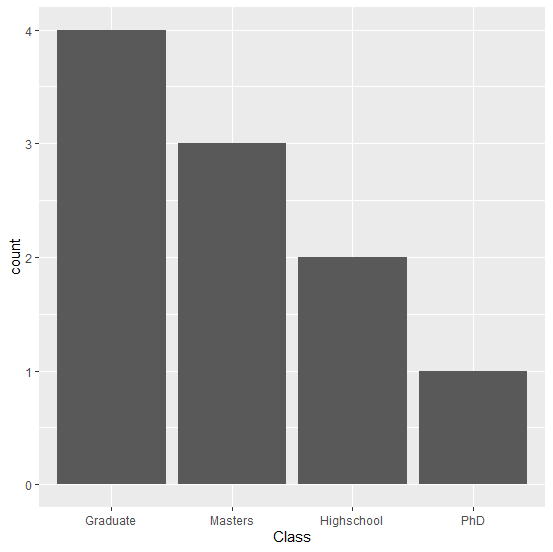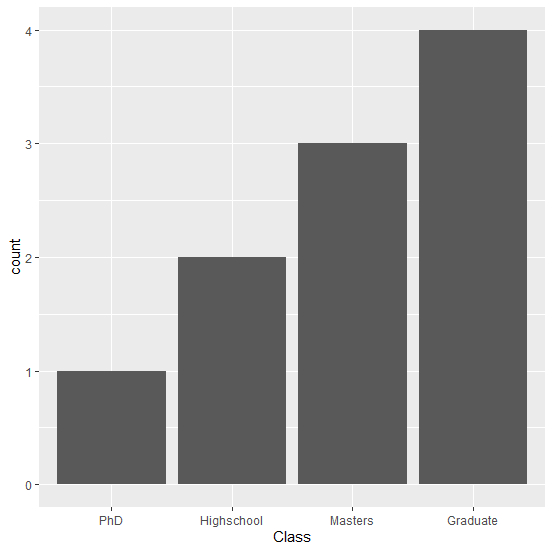# How to change the order of bars in bar chart in R?

This can be done by setting the levels of the variable in the order we want.

## Example

> data <- data.frame(Class=c("Highschool","Highschool","Graduate","Graduate",
"Graduate","Graduate","Masters","Masters","Masters","PhD"))

Setting the levels in decreasing order

> data <- within(data,
Class <- factor(Class,
levels=names(sort(table(Class),
decreasing=TRUE))))
> library(ggplot2)
> ggplot(data, aes(x = Class)) + geom_bar()Setting the levels in increasing order

> data <- within(data,
Class <- factor(Class,
levels=names(sort(table(Class),
decreasing=TRUE))))
> ggplot(data, aes(x = Class)) + geom_bar()